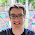## Friday, 6 May 2011

### Data simulation using concurrent independent Markov chains of latent variables

As explained in previous posts, we can model the conditional probabilities of appliance states and aggregate power readings using a Markov chain of latent variables:

where:
• Zt - discrete latent variable at time t (appliance state)
• Xt - continuous observed variable at time t (power reading)

This model can be manually trained by specifying the model parameters:

• pi - probability density of z1 (probabilities of initial appliance states)
• A - appliance transition probability matrix
• phi - emission density (probability density of observed variable given a hidden variable)
The model can then be used to simulate the states of an appliance and its power demand over time.

This is trivially extended to multiple appliances by using multiple concurrent Markov chains. However, this makes the assumption that appliance operations are independent of each other. Aggregate readings would be calculated by summing over each chain's observed variable for each time slice. Gaussian noise can be added to such a model using an additional Markov chain of latent variables, in which the latent variable is discrete and has only one state and the emission density represents the noise. This is equivalent to the factorial hidden Markov model below:
where:
• Zt (1...n) - discrete latent variable at time t (appliance state)
• Zt (0) - discrete latent variable at time t (meter noise source)
• Xt - continuous observed variable at time t (power reading)

1.Hey Oliver,
I am curious as to if you've tried inference on your factorial HMMs, and what your experience was on computational complexity was.

Nice meeting you at the NILM conference btw. Hope you got to try some yuengling.

-Suman

2.Hi Suman,

I have tried inference on factorial HMMs but only on toy data sets (5 appliances) which I wrote up for my 9-month report, available at the bottom of this page:

With regard to computational complexity, exact inference isn't tractable for realistic numbers of appliances (10-20). These three papers have demonstrated how approximate inference can work over such models:

http://www.cs.uiuc.edu/~hanj/pdf/sdm11_hkim.pdf

http://people.csail.mit.edu/kolter/lib/exe/fetch.php?media=pubs:kolter-kddsust11.pdf

http://people.csail.mit.edu/kolter/lib/exe/fetch.php?media=pubs:kolter-aistats12.pdf

And with regard to Yuengling, I did try some at the restaurant on Schenley Plaza. Thanks for the recommendation!

3.